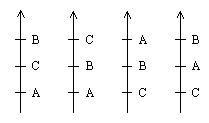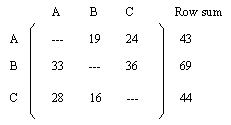## Project 8: Vote Matrix (Table)

The diagram below shows an election where 52 voters ranked three candidates.Each voter has indicated his/her preferences, and the "arrow" on the left is interpreted to mean that 12 voters preferred B to C, B to A and C to A. This notation can be extended to allow voters who are indifferent between two or more candidates. (For simplicity, for most of the discussion below we exclude elections where voters are indifferent between two or more candidates.)

We can construct a matrix (table) to represent this election as follows: Label the rows and columns of the matrix with the candidates' names. The entry in the row labeled i and the column labeled j is the number of voters who preferred candidate i to candidate j.The row sums are of interest because they allow one to use the vote matrix to compute the winner of a popular method of deciding elections called the Borda Count. In the Borda Count candidates are given credit in the form of points for how high up on a voter's preference schedule a candidate appears. The number of points a candidate would get would be the number of candidates below him/her on the preference schedule. For example, to compute the Borda Count for candidate B in the election above, starting with the preference schedule which received 12 votes, B would get: 12 votes times 2 points + 16 votes times 1 point + 19 votes times 1 point + 5 votes times 2 points =

12(2) + 16(1) + 1(19) + 5(2) = 24 + 16 + 19 + 10 = 69.

Note that this is exactly the row sum of the second (B) row of the vote matrix.

Problem

Given an election, it is simple to compute its vote matrix. However, given a vote matrix, one can ask the question of whether or not there is some election which gives rise to the vote matrix. The problem is: given a vote matrix which is 3x3 with "blank entries" on the diagonals, give an algorithm (mechanical procedure) for constructing an election, if one exists, which has the given matrix as its vote matrix.

Extensions

1. Consider the same question for matrices which arise from elections where there are n (rather than 3) candidates.

2. Consider the problem when ties between candidates in the preference schedules of individual voters are permitted.

References:

1. Garfunkel, S., (project director), For All Practical Purposes, 4th ed., W. H. Freeman, New York, 1997.

2. Malkevitch, J. and W. Meyer, Graphs, Models and Finite Mathematics, Prentice-Hall, Englewood Cliffs, 1974.

3. Malkevitch, J. and G. Froelich, The Mathematical Theory of Elections, COMAP, Lexington, 1988.

4. Straffin, P., Topics in the Theory of Voting, Birkhäuser Boston, Boston, 1980.

Joseph Malkevitch
Department of Mathematics and Computing
York College (CUNY)
Jamaica, New York 11451-0001
email: malkevitch@york.cuny.edu
(Comments and results related to the project above are welcome.)

Acknowledgements
Some of this work was prepared with partial support from the National Science Foundation (Grant Number: DUE 9555401) to the Long Island Consortium for Interconnected Learning (administered by SUNY at Stony Brook, Alan Tucker, Project Director).